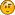###Author Topic: Calculate Volume?  (Read 2618 times)

####Alexei_B

• byte
•• Posts: 4##### Calculate Volume?
« on: December 12, 2007, 03:08:57 pm »
I suspect this simple question may be difficult to answer, but how do I calculate the volume of a closed 3-d shape?   Obviously simple geometric primitives like cubes, rectangles, spheres, cones etc. all have known formulae.

Even shapes bounded by known equations describing planes can have volumes found via 3d integration methods.

But what if I need the volume of a more complex mesh/object representing a simple house for example?

My only thought is that 2d areas can be calculated by iterative methods - literally filling a space with tiny units and counting them - something like how the fill or paint bucket tools work in drawing programmes.  Perhaps this could be extended to a 3d solution?

####EgonOlsen

••• Posts: 12020##### Re: Calculate Volume?
« Reply #1 on: December 12, 2007, 10:18:21 pm »
I have no idea...sounds like a very complicated problem. Your approach may work but sounds time consuming.####Hrolf

• int
•• Posts: 84##### Re: Calculate Volume?
« Reply #2 on: December 13, 2007, 06:30:32 pm »
If time isn't a problem you could use the method they use in stone carving;

Pseudocode;
modelVolume=BBoxVolume;
For each face of the bounding box (ie +X by YZ, -X by YZ, +Z by XY, &c)
scan the BBox face in smallUnits (use 2 nested for loops)
use dist=calcMinDistance to get distance from the point on the BBox face to the model
modelVolume-=small unit * smallUnit * dist

The smaller smallUnit is, the slower it is but the closer the result is to the actual volume...

This method only really works properly on convex models!